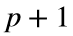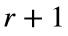# Problem 51798. Label primes with the Erdos-Selfridge classification

Erdos and Selfridge developed a scheme for classifying a prime number p: If the only factors ofare 2 and 3, then put p in class 1. Otherwise, find the largest class r of the factors ofand put p in class.
For example, 11 is in class 1 because 12 has prime factors 2, 2, and 3. The number 43 is in class 2 because 44 has prime factors 2, 2, and 11, and all of those are in class 1. All Mersenne primes are in class 1, and all ugly numbers are in classes 1 or 2.
Write a function to classify prime numbers using this scheme.

### Solution Stats

80.0% Correct | 20.0% Incorrect
Last Solution submitted on May 06, 2023

### Community Treasure Hunt

Find the treasures in MATLAB Central and discover how the community can help you!

Start Hunting!